# designHalfbandFIR

Design and implement halfband FIR filter

Since R2023b

## Syntax

``B = designHalfbandFIR``
``B = designHalfbandFIR(Name=Value)``

## Description

example

````B = designHalfbandFIR` designs a halfband FIR equiripple filter with the filter order of 24 and the transition width of 0.1. `B` is a vector of halfband FIR coefficients of length 25.The System object™ argument is `false` by default. To implement the filter, assign the filter coefficients in `B` to one of the supported System objects.```

example

````B = designHalfbandFIR(Name=Value)` specifies options using one or more name-value arguments.For example, ```B = designHalfbandFIR(FilterOrder=30,TransitionWidth=0.2,DesignMethod="kaiser",SystemObject=true)``` designs a halfband FIR filter with the filter order of 30 and transition width of 0.2 by using the Kaiser window design method. As the `SystemObject` argument is `true`, the function designs and implements the halfband FIR filter. `B` is a `dsp.FIRFilter` System object in this case.When you specify only a partial list of filter parameters, the function designs the filter by setting the other design parameters to their default values.The function supports three design methods. Each design method supports a specific set of design combinations. For more information, see `DesignMethod`.This function supports code generation under certain conditions. For more information, see Code Generation.```

## Examples

collapse all

Design an equiripple FIR halfband filter with the order of 24 and a transition width of 0.1 using the `designHalfbandFIR` function. Assign the filter coefficients to a `dsp.FIRFilter` System object.

```b = designHalfbandFIR(FilterOrder=24,DesignMethod='equiripple'); hbFIR = dsp.FIRFilter(b);```

Create a `dsp.DynamicFilterVisualizer` object and visualize the magnitude response of the filter.

```dfv = dsp.DynamicFilterVisualizer(NormalizedFrequency=true); dfv(hbFIR);```Create a `spectrumAnalyzer` object to visualize the spectra of the input and output signals.

```scope = spectrumAnalyzer(SampleRate=2, PlotAsTwoSidedSpectrum=false,... ChannelNames=["Input Signal","Filtered Signal"]); ```

Stream in random data and filter the signal using the FIR halfband filter.

```for i = 1:1000 x = randn(1024, 1); y = hbFIR(x); scope(x,y); end```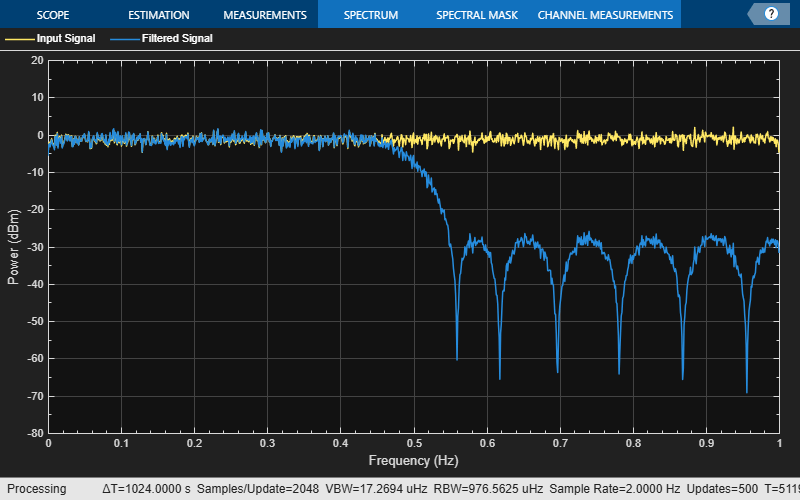Design an equiripple FIR halfband interpolator object of order 48 using the `designHalfbandFIR` function. Set the `Verbose` argument to `true`.

```hbFIR = designHalfbandFIR(FilterOrder=48,SystemObject=true,... Structure='interp',Verbose=true)```
```designHalfbandFIR(FilterOrder=48, TransitionWidth=0.1, DesignMethod="equiripple", Passband="lowpass", Structure="interp", SystemObject=true) ```
```hbFIR = dsp.FIRHalfbandInterpolator with properties: Specification: 'Coefficients' Numerator: [0 -0.0082 0 0.0079 0 -0.0116 0 0.0165 0 -0.0227 0 0.0309 0 -0.0419 0 0.0571 0 -0.0800 0 0.1193 0 -0.2073 0 0.6350 1 0.6350 0 -0.2073 0 0.1193 0 -0.0800 0 0.0571 0 -0.0419 0 0.0309 0 -0.0227 0 0.0165 0 -0.0116 ... ] (1x49 double) FilterBankInputPort: false Use get to show all properties ```

Create a `dsp.DynamicFilterVisualizer` object and visualize the magnitude response of the filter.

```dfv = dsp.DynamicFilterVisualizer(NormalizedFrequency=true); dfv(hbFIR);```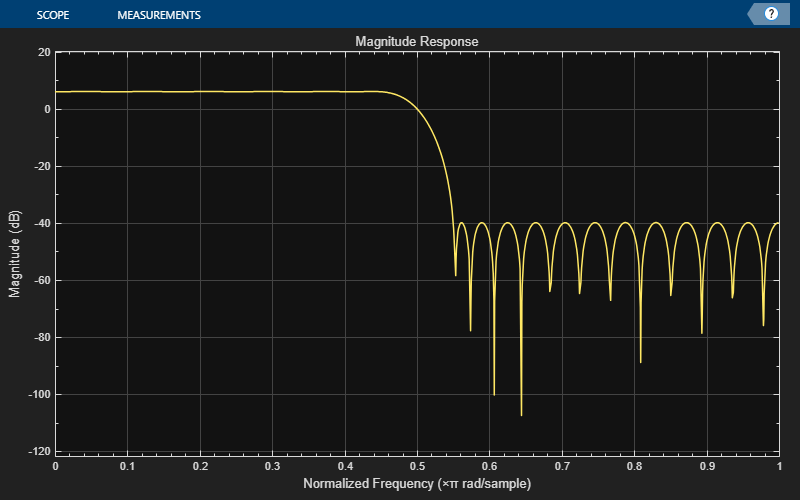The input is a cosine wave with an angular frequency of $\frac{\pi }{4}$ radians/sample.

`input = cos(pi/4*(0:39)');`

Interpolate the cosine signal using the FIR halfband interpolator.

`output = hbFIR(input);`

Plot the original and interpolated signals. In order to plot the two signals on the same plot, you must account for the output delay introduced by the FIR halfband interpolator and the scaling introduced by the filter. Use the `outputDelay` function to compute the `delay` value introduced by the interpolator. Shift the output by this delay value.

Visualize the input and the resampled signals. The input and output values coincide every other sample due to the interpolation factor of 2.

`[delay,FsOut] = outputDelay(hbFIR,FsIn=1)`
```delay = 12 ```
```FsOut = 2 ```
```nInput = (0:length(input)-1); tOutput = (0:length(output)-1)/FsOut-delay; stem(tOutput,output,'filled',MarkerSize=4); hold on; stem(nInput,input); hold off; xlim([-5,20]) legend('Interpolated by 2','Input signal','Location','best');```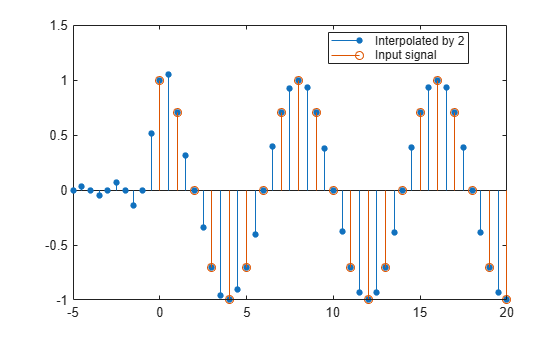Design an equiripple FIR halfband decimator object of order 48 using the `designHalfbandFIR` function. Set the `Verbose` argument to `true`.

```hbFIR = designHalfbandFIR(FilterOrder=48,SystemObject=true,... Structure='decim',Verbose=true)```
```designHalfbandFIR(FilterOrder=48, TransitionWidth=0.1, DesignMethod="equiripple", Passband="lowpass", Structure="decim", SystemObject=true) ```
```hbFIR = dsp.FIRHalfbandDecimator with properties: Main Specification: 'Coefficients' Numerator: [0 -0.0041 0 0.0040 0 -0.0058 0 0.0082 0 -0.0114 0 0.0155 0 -0.0209 0 0.0286 0 -0.0400 0 0.0597 0 -0.1037 0 0.3175 0.5000 0.3175 0 -0.1037 0 0.0597 0 -0.0400 0 0.0286 0 -0.0209 0 0.0155 0 -0.0114 0 0.0082 0 -0.0058 0 0.0040 0 -0.0041 0] Use get to show all properties ```

Create a `dsp.DynamicFilterVisualizer` object and visualize the magnitude response of the filter.

```dfv = dsp.DynamicFilterVisualizer(NormalizedFrequency=true); dfv(hbFIR);```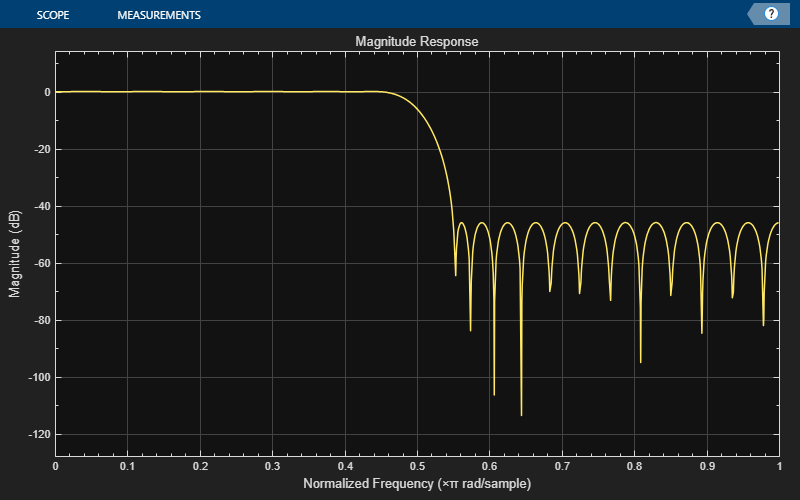The input is a cosine wave with an angular frequency of $\frac{\pi }{4}$ radians/sample.

`input = cos(pi/4*(0:39)');`

Decimate the cosine signal using the FIR halfband decimator.

`output = hbFIR(input);`

Plot the original and decimated signals. In order to plot the two signals in the same plot, you must account for the output delay of the FIR halfband decimator and the scaling introduced by the filter. Use the `outputDelay` function to compute the `delay` introduced by the decimator. Shift the output by this delay value.

Visualize the input and the resampled signals. After a short transition, the output converges to a cosine of frequency $\frac{\pi }{2}$, as expected, which is twice the frequency of the input signal, $\frac{\pi }{4}$. Due to the decimation factor of 2, the output samples coincide with every other input sample.

`[delay,FsOut] = outputDelay(hbFIR,FsIn=1)`
```delay = 24 ```
```FsOut = 0.5000 ```
```nInput = (0:length(input)-1); tOutput = (0:length(output)-1)/FsOut-delay; stem(tOutput,output,'filled',MarkerSize=4); hold on; stem(nInput,input); hold off; xlim([-10,15]) legend('Decimated by 2','Input signal','Location','best');```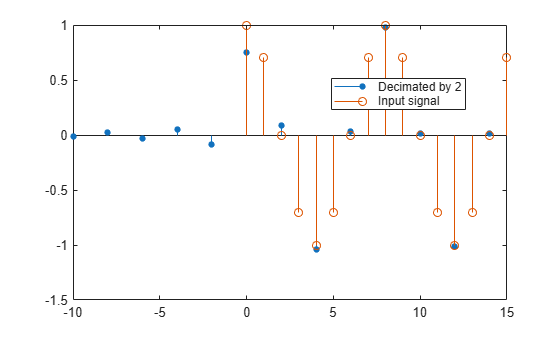## Input Arguments

collapse all

### Name-Value Arguments

Specify optional pairs of arguments as `Name1=Value1,...,NameN=ValueN`, where `Name` is the argument name and `Value` is the corresponding value. Name-value arguments must appear after other arguments, but the order of the pairs does not matter.

Example: `designHalfbandFIR(FilterOrder=30,TransitionWidth=0.3,Passband='lowpass')`

Order of the halfband FIR filter, N, specified as an even nonnegative integer.

Data Types: `single` | `double` | `int8` | `int16` | `int32` | `int64` | `uint8` | `uint16` | `uint32` | `uint64`

Transition width of the halfband FIR filter, TW, specified as a normalized scalar in the range (`0`,`1`].

Data Types: `single` | `double`

Stopband attenuation of the halfband FIR filter, Ast, specified as a positive scalar in dB units.

Data Types: `single` | `double`

Window design method, specified as one of these options:

• `'auto'` –– When you do not specify a window design method or specify `'auto'`, the function automatically chooses the best design method for the given specification parameters.

• `'kaiser'` –– Kaiser method supports all the design specification combinations the function supports.

Here is the list:

• `'equiripple'` –– Equiripple method supports all the design specification combinations.

• `'ls'` –– Least squares method supports the filter order (N) and transition width (TW) specifications.

Data Types: `char` | `string`

Passband frequency response, specified as one of these:

• `'lowpass'` –– This option supports all three filter structures when you set `SystemObject` to `true`.

• `'highpass'` –– This option supports only the `'single-rate'` filter structure when you set `SystemObject` to `true`.

Data Types: `char` | `string`

Option to create System object, specified as one of these:

Data Types: `logical`

Filter structure, specified as one of these:

• `'single-rate'` –– When you set `SystemObject` to:

• `true` –– The function returns a `dsp.FIRFilter` object.

• `false` –– The function returns a vector of halfband FIR filter coefficients. The center coefficient is 0.5.

• `'decim'` –– When you set `SystemObject` to:

• `true` –– The function returns a `dsp.FIRHalfbandDecimator` object.

• `false` –– The function returns a vector of halfband FIR filter coefficients. The center coefficient is 0.5.

• `'interp'` –– When you set `SystemObject` to:

• `true` –– The function returns a `dsp.FIRHalfbandInterpolator` object.

• `false` –– The function returns a vector of halfband FIR filter coefficients. The filter coefficients are scaled by 2 and the center coefficient is 1.0.

Data Types: `char` | `string`

Option to print the entire function call in MATLAB, specified as one of these:

• `false` –– The function does not print the function call.

• `true` –– The function prints the entire function call including the default values of the `Name=Value` arguments that you did not specify when calling the function.

Use this argument to view all the values used by the function to design and implement the filter.

Data Types: `logical`

## Output Arguments

collapse all

Halfband FIR filter coefficients or filter object, returned as one of these:

• Row vector –– The function returns a row vector of length `FilterOrder` + 1 when you set the `SystemObject` argument to `false`.

• `dsp.FIRFilter` System object –– The function returns a `dsp.FIRFilter` object when you set `Structure` to `'single-rate'` and the `SystemObject` argument to `true`.

• `dsp.FIRHalfbandDecimator` System object –– The function returns a `dsp.FIRHalfbandDecimator` object when you set `Structure` to `'decim'` and the `SystemObject` argument to `true`.

• `dsp.FIRHalfbandInterpolator` System object –– The function returns a `dsp.FIRHalfbandInterpolator` object when you set `Structure` to `'interp'` and the `SystemObject` argument to `true`.

Data Types: `single` | `double`

collapse all

### Halfband Filters

An ideal lowpass halfband filter is given by

`$h\left(n\right)=\frac{1}{2\pi }{\int }_{-\pi /2}^{\pi /2}{e}^{j\omega n}d\omega =\frac{\mathrm{sin}\left(\frac{\pi }{2}n\right)}{\pi n}.$`

An ideal filter is not realizable. However, the impulse response of an ideal lowpass filter possesses some important properties that are required in a realizable approximation. The impulse response of an ideal lowpass halfband filter is:

• Equal to 0 for all even-indexed samples.

• Equal to 1/2 at n=0 as shown by L'Hôpital's rule on the continuous-valued equivalent of the discrete-time impulse response

The ideal highpass halfband filter is given by

`$g\left(n\right)=\frac{1}{2\pi }{\int }_{-\pi }^{-\pi /2}{e}^{j\omega n}d\omega +\frac{1}{2\pi }{\int }_{\pi /2}^{\pi }{e}^{j\omega n}d\omega .$`

Evaluating this integral yields the impulse response

`$g\left(n\right)=\frac{\mathrm{sin}\left(\pi n\right)}{\pi n}-\frac{\mathrm{sin}\left(\frac{\pi }{2}n\right)}{\pi n}.$`

The impulse response of an ideal highpass halfband filter is:

• Equal to 0 for all even-indexed samples

• Equal to 1/2 at n=0

The FIR halfband filter uses a causal FIR approximation of the ideal halfband response. The approximation is based on minimizing the ${\ell }^{\infty }$ norm of the error (minimax). See Algorithms for more information.

### Kaiser Window

The `designHalfbandFIR` function computes the coefficients of a Kaiser window using the equation

`$w\left(n\right)=\frac{{I}_{0}\left(\beta \sqrt{1-{\left(\frac{n-N/2}{N/2}\right)}^{2}}\right)}{{I}_{0}\left(\beta \right)},\text{ }\text{ }0\le n\le N,$`

where I0 is the zeroth-order modified Bessel function of the first kind.

To obtain a Kaiser window that represents an FIR filter with stopband attenuation of α dB, use this β.

`$\beta =\left\{\begin{array}{ll}0.1102\left(\alpha -8.7\right),\hfill & \alpha >50\hfill \\ 0.5842{\left(\alpha -21\right)}^{0.4}+0.07886\left(\alpha -21\right),\hfill & 50\ge \alpha \ge 21\hfill \\ 0,\hfill & \alpha <21\hfill \end{array}$`

The filter order n is given by

`$n=\frac{\alpha -7.95}{2.285\left(\Delta \omega \right)},$`

where Δω is the transition width.

## Algorithms

collapse all

### Filter Design Method

The `designHalfbandFIR` function uses the equiripple, Kaiser, or the least-squares linear phase window method to design the FIR halfband filter. When the design constraints are tight, such as very high stopband attenuation or very narrow transition width, use the Kaiser window method. If you are not sure of which method to use, set the design method to `"auto"`. In this mode, the algorithm automatically chooses a design method that optimally meets the specified filter constraints.

Halfband Equiripple Design

In the equiripple method, the algorithm uses a minimax (minimize the maximum error) FIR design to design a fullband linear phase filter with the desired specifications. The algorithm upsamples a fullband filter to replace the even-indexed samples of the filter with zeros and creates a halfband filter. It then sets the filter tap corresponding to the group delay of the filter in samples to 1/2. This yields a causal linear-phase FIR filter approximation to the ideal halfband filter defined in Halfband Filters. See  for a description of this filter design method using the Remez exchange algorithm. As you can design a filter using this approximation method with a constant ripple both in the passband and stopband, the filter is also known as the equiripple filter.

Halfband Kaiser Window Design

In the Kaiser window method, the algorithm first truncates the ideal halfband filter defined in Halfband Filters, then it applies a Kaiser window defined in Kaiser Window. This yields a causal linear-phase FIR filter approximation to the ideal halfband filter.

Least-Squares Linear-Phase FIR Filter Design

The function designs a linear-phase FIR filter by minimizing the weighted, integrated, and squared error between the magnitude response of the ideal halfband filter and the filter designed for the given set of specifications. For more information, see .

### Filter Implementation

To implement the designed filter, set `SystemObject` to `true` and `Structure` to:

For more information on how the objects implement the filters, see the Algorithms section in the corresponding object reference page.

 Harris, F.J. Multirate Signal Processing for Communication Systems, Prentice Hall, 2004, pp. 208–209.

 Orfanidis, Sophocles J. Introduction to Signal Processing. Upper Saddle River, NJ: Prentice-Hall, 1996.

 Oppenheim, Alan V., Ronald W. Schafer, and John R. Buck. Discrete-Time Signal Processing. Upper Saddle River, NJ: Prentice Hall, 1999.

 Parks, Thomas W., and C. Sidney Burrus. Digital Filter Design. Hoboken, NJ: John Wiley & Sons, 1987, pp. 54–83.